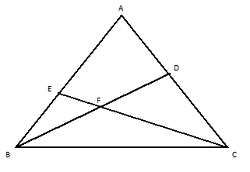GMAT Question of the Day - Daily to your Mailbox; hard ones only

 It is currently 20 Jun 2019, 12:28GMAT Club Daily Prep

Thank you for using the timer - this advanced tool can estimate your performance and suggest more practice questions. We have subscribed you to Daily Prep Questions via email.

Customized
for You

we will pick new questions that match your level based on your Timer History

Track

every week, we’ll send you an estimated GMAT score based on your performance

Practice
Pays

we will pick new questions that match your level based on your Timer History

Not interested in getting valuable practice questions and articles delivered to your email? No problem, unsubscribe here.Mastering Important Concept on Triangles – III - Question #1

Author Message
TAGS:

Hide Tags

e-GMAT RepresentativeD
Joined: 04 Jan 2015
Posts: 2892
Mastering Important Concept on Triangles – III - Question #1  [#permalink]

Show Tags

1
700:00

Difficulty:65% (hard)

Question Stats:68% (02:40) correct32% (02:53) wrongbased on 290 sessions

HideShow timer Statistics

Mastering Important Concept on Triangles – III - Question #1

In the given figure, BD is the median of triangle ABC and the lines BD and CE intersect at point F. If the area of triangle BFC is $$6cm^2$$ and area of the quadrilateral FEAD is $$18cm^2$$. Find the area of triangle FDC, if the ratio of the area of triangle FDC and triangle FEB is $$3:2$$.a. $$12 cm^2$$
b. $$18 cm^2$$
c. $$24 cm^2$$
d. $$36 cm^2$$
e. $$42 cm^2$$

Key concepts on Triangles are explained in detail in the following posts:

1. Mastering Important Concepts Tested By GMAT in Triangle - I

2. Mastering Important Concepts Tested By GMAT in Triangle - II

3.Mastering Important Concepts Tested By GMAT in Triangle - III

Detailed solution will be posted soon.Thanks,
Saquib
Quant Expert
e-GMAT_________________

Originally posted by EgmatQuantExpert on 11 Dec 2016, 08:29.
Last edited by EgmatQuantExpert on 15 Dec 2016, 23:50, edited 1 time in total.
e-GMAT RepresentativeD
Joined: 04 Jan 2015
Posts: 2892
Re: Mastering Important Concept on Triangles – III - Question #1  [#permalink]

Show Tags

4
2
Hey,

PFB the official solutionIt is given that BD is the median of the triangle ABC.

From the given information we can conclude that:
Area of Triangle ABD = Area of Triangle BDC
Which is equal to,
Area of Triangle FEB + Area of Quadrilateral FEAD = Area of Triangle BFC + Area of Triangle FDC…. (i)

We are also given the following information –
• Area of BFC = $$6 cm^2$$
• Area of Quadrilateral FEAD = $$18cm^2$$
• And area of FDC: Area of FEB = 3: 2
o Let us consider the area of FDC and FEB to be $$3x$$ and $$2x$$ respectively.

Let us substitute the above values in equation (i)
Area of Triangle FEB + Area of Quadrilateral FEAD = Area of Triangle BFC + Area of Triangle FDC
o $$2x + 18 = 6 + 3x$$
o $$x = 12 cm^2$$
• Thus the Area of Triangle FDC = $$3x = 36 cm^2$$

Hence the correct answer option is D

Thanks,
Saquib
Quant Expert
e-GMAT
_________________

Originally posted by EgmatQuantExpert on 11 Dec 2016, 08:31.
Last edited by EgmatQuantExpert on 15 Dec 2016, 23:49, edited 1 time in total.
General Discussion
Current StudentB
Joined: 07 Mar 2015
Posts: 109
Location: India
Concentration: General Management, Operations
GMAT 1: 590 Q46 V25GPA: 3.84
WE: Engineering (Energy and Utilities)
Re: Mastering Important Concept on Triangles – III - Question #1  [#permalink]

Show Tags

1
Since BD is median , The area of triangle BDA= Area of triangle BDC

The area of triangle BDA= Area of triangle BDC

Area of BFE+ Area EFAD = Area BFC+ Area CFD

2x + 18 = 3x+ 6

X= 12.

we need 3x = 36 cm^2
e-GMAT RepresentativeD
Joined: 04 Jan 2015
Posts: 2892
Mastering Important Concept on Triangles – III - Question #1  [#permalink]

Show Tags

Hey Everyone,

The official solution has been posted. Please go through the solution once. In case of any doubts please feel to askThanks,
Saquib
Quant Expert
e-GMAT
_________________
InternB
Joined: 07 Dec 2016
Posts: 39
Mastering Important Concept on Triangles – III - Question #1  [#permalink]

Show Tags

As BD is the median , it divides triangle ABC into two triangles of equal area.

Area of triangle ABD = Area of triangle BDC
Area of triangle BFE + Area of quadilateral EAFD = Area of triangle BFC + Area of triangle FDC ........(1)

Also, Area of FDC/Area of FEB = 3/2
which gives us , Area of FEB = 2*Area of FDC / 3

Substituting above relation in our equation (1)

2*Area of FDC / 3 +Area of EFAD = Area of BFC + Area of FDC
Area of FDC/3 = Area of EFAD - Area of BFC
Area of FDC = 3( 18-6)
Area of FDC = 36 cm^2

Option D
Non-Human UserJoined: 09 Sep 2013
Posts: 11399
Re: Mastering Important Concept on Triangles – III - Question #1  [#permalink]

Show Tags

Hello from the GMAT Club BumpBot!

Thanks to another GMAT Club member, I have just discovered this valuable topic, yet it had no discussion for over a year. I am now bumping it up - doing my job. I think you may find it valuable (esp those replies with Kudos).

Want to see all other topics I dig out? Follow me (click follow button on profile). You will receive a summary of all topics I bump in your profile area as well as via email.
_________________Re: Mastering Important Concept on Triangles – III - Question #1   [#permalink] 15 Sep 2018, 11:34
Display posts from previous: Sort by

Mastering Important Concept on Triangles – III - Question #1